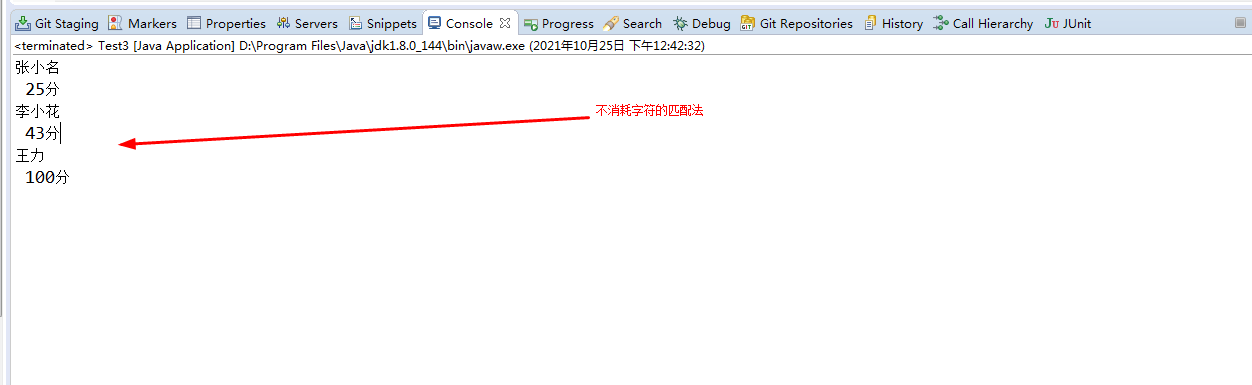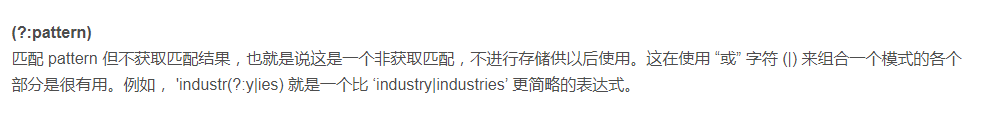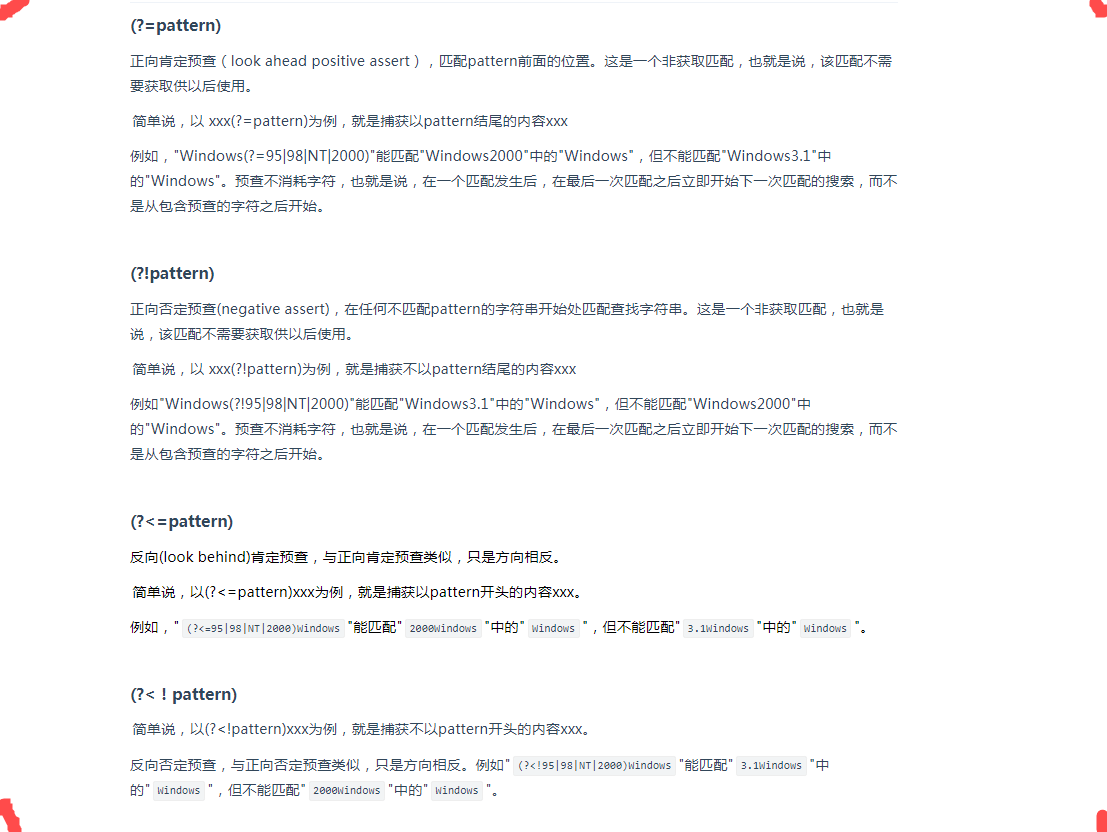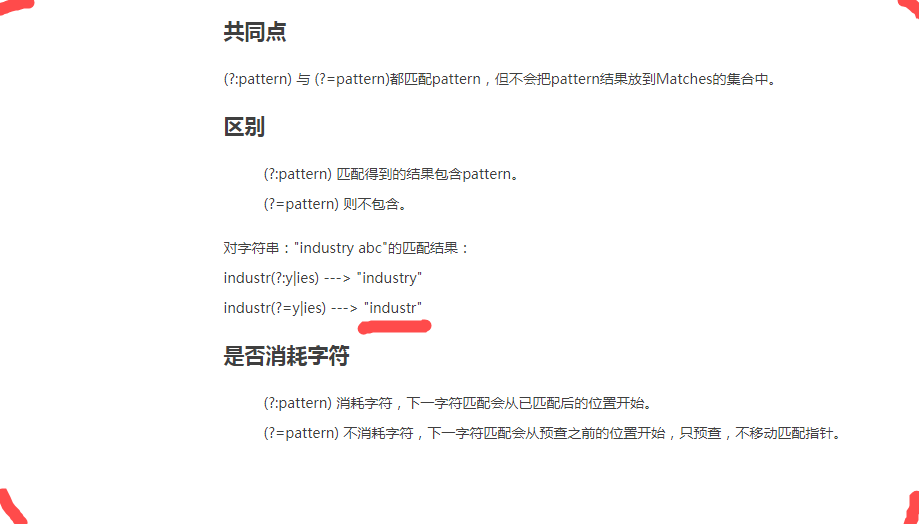# java正则-取出指定字符串之间的内容

2021-10-29    留住此刻

``````public static void main(String[] args) {
/Pattern p = Pattern.compile("\@(.*?)\:");//正则表达式，取=和|之间的字符串，不包括=和|

String filetext = "abc张小名abc 25分abc李小花abc 43分abc王力abc 100分abc";
Pattern p = Pattern.compile("abc(.*?)abc");

Matcher m = p.matcher(filetext);
while(m.find()) {
System.out.println(m.group());//m.group(1)不包括这两个字符

}``````

``````public static void main(String[] args) {

String filetext = "abc张小名abc 25分abc李小花abc 43分abc王力abc 100分abc";
//Pattern p = Pattern.compile("abc(.*?)abc");//正则表达式 会消耗字符串abc
Pattern p = Pattern.compile("(?<=abc)(.*?)(?=abc)");//不消耗字符串abc

Matcher m = p.matcher(filetext);
while(m.find()) {
System.out.println(m.group(1));//m.group(1)不包括这两个字符

}
}``````(?:pattern) 与 (?=pattern)也有一些区别，主要在于消耗字符、是否输出匹配结果方面，如下图已经说得很清楚了▍相关推荐